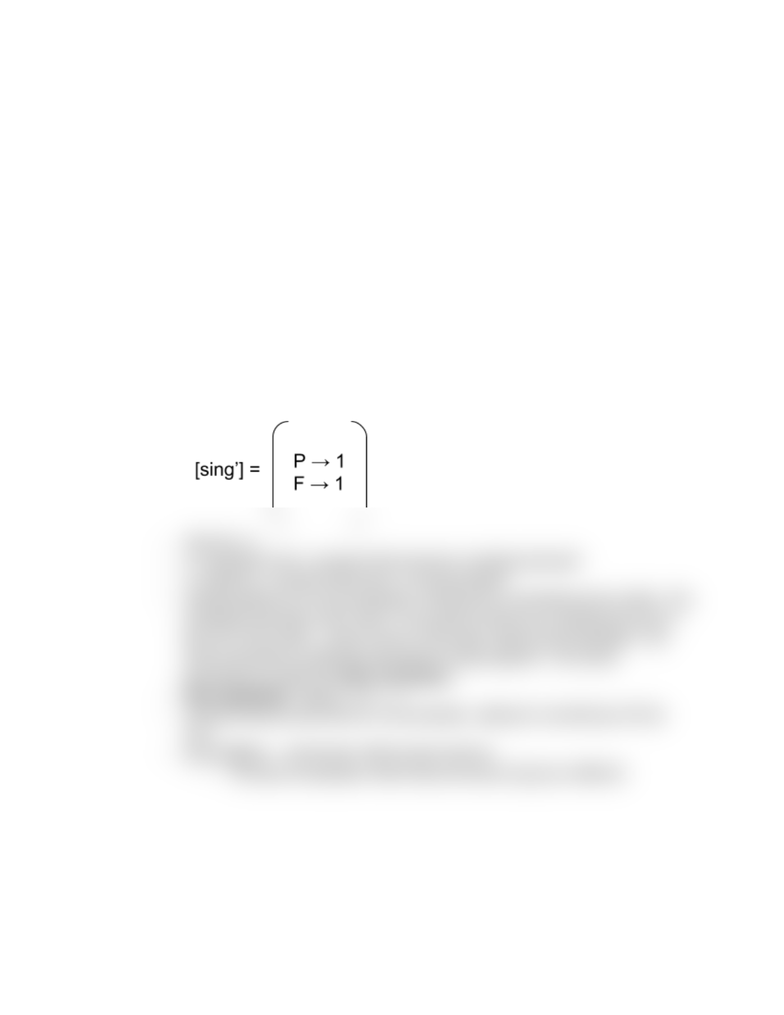Class Notes (1,200,000)
US (500,000)
UCLA (10,000)
LING (100)
Lecture 4

# LING 165C Lecture 4:

Department
Linguistics
Course Code
LING 165C
Professor
Dylan Bumford
Lecture
4

This preview shows half of the first page. to view the full 2 pages of the document.1/28/19 Lecture 5
- Review
- Predicates are functions that take in arguments (i.e pred’(c)(John’),
chase’(Mary’)(John’)
)
- Variable binding operators (, ), can be used on any sentence. Where we
evaluate the sentence under certain conditions.
- i.e y [ x [ use’(x)(y) ] ]. The whole expression doesn’t need to know
what x or y is.
- Variables & constant → entities; predicates → functions; sentences → truth
conditions
- [P(𝛕)]g = [P]g([𝛕]g) used to find out the denotation of the predicate
- i.e [v[φ])]g = 1 iff [φ]g = 1 for every g’ like g except for φ
- Lambda Calculus
- [ λv [φ]]g = F where F(a) = [φ]^(gr→ a).
- (gr→ a) = an assignment just like g except v is mapped to A
- Example: [sing’] is a predicate that denotes a function from entities to truth
values. As with all predicates and functions, we just assume we know what this
predicate sing.
- λv[p’(v)] = p
- i.e λx[chase’(x)(x)] = property that someone is chasing themself
- i.e chase’(x) = property that some x is being chased
- λx[sing’(x)](john) is a new expression combined by a predicate and an entity. This
expression denotes a truth value. The property holds up by λx[sing’(x)] is true if
and only if the entity x, which is john in this case, holds up this predicate. This
whole expression is basically equivalent to [sing’(x)](john). This whole
equivalence is called the beta-conversion.
-Beta-equivalent: λv[p](ε) = p/ v ε
- Lambda-calculus just returns a new predicate, waiting for something to fill the
void.
- [λx[λy[x]]]([y]) gives back what is given second
- The above expression when beta-converted (λy[y]) are different